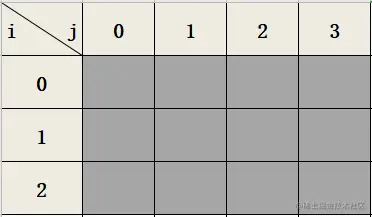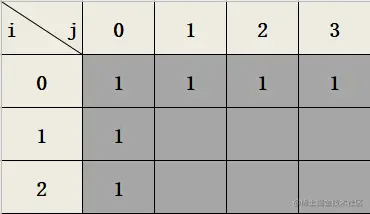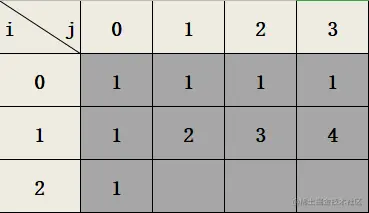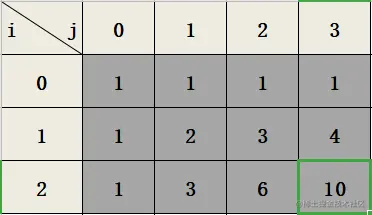# 前言输入： m = 3, n = 7

输入： m = 3, n = 2

1. 向右 -> 向下 -> 向下
2. 向下 -> 向下 -> 向右
3. 向下 -> 向右 -> 向下

输入： m = 7, n = 3

# 一、思路

不同路径这一题中有两个重要的信息：

• 每次只能向右向下
• 需要计算的结果为不同路径的数量

## 图解动态规划

1. 初始化二维数组 dp 如下图所示1. 先填充第一行和第一列，值都为 11. 从第二行开始遍历，当 i=1 时，根据状态转移方程填充此行1. i=2 时，根据状态转移方程填充此行1. 返回 dp 的结果 10 即可

# 二、实现

### 实现代码

    /**
* 动态规划
*/
public int uniquePaths(int m, int n) {
int[][] dp = new int[m][n];
// 初始化第一行和第一列
for (int i=0; i<n; i++) {
dp[i] = 1;
}
for (int i=0; i<m; i++) {
dp[i] = 1;
}
// 遍历dp（从  开始）
for (int i=1; i<m; i++) {
for (int j=1; j<n; j++) {
dp[i][j] = dp[i-1][j] + dp[i][j-1];
}
}
return dp[m-1][n-1];
}

### 测试代码

    public static void main(String[] args) {
new Number62().uniquePaths(3, 4);
}

### 结果